JEE  >  BITSAT Maths Test - 2

# BITSAT Maths Test - 2

Test Description

## 45 Questions MCQ Test BITSAT Mock Tests Series & Past Year Papers | BITSAT Maths Test - 2

BITSAT Maths Test - 2 for JEE 2023 is part of BITSAT Mock Tests Series & Past Year Papers preparation. The BITSAT Maths Test - 2 questions and answers have been prepared according to the JEE exam syllabus.The BITSAT Maths Test - 2 MCQs are made for JEE 2023 Exam. Find important definitions, questions, notes, meanings, examples, exercises, MCQs and online tests for BITSAT Maths Test - 2 below.
Solutions of BITSAT Maths Test - 2 questions in English are available as part of our BITSAT Mock Tests Series & Past Year Papers for JEE & BITSAT Maths Test - 2 solutions in Hindi for BITSAT Mock Tests Series & Past Year Papers course. Download more important topics, notes, lectures and mock test series for JEE Exam by signing up for free. Attempt BITSAT Maths Test - 2 | 45 questions in 60 minutes | Mock test for JEE preparation | Free important questions MCQ to study BITSAT Mock Tests Series & Past Year Papers for JEE Exam | Download free PDF with solutions
 1 Crore+ students have signed up on EduRev. Have you?
BITSAT Maths Test - 2 - Question 1

### The area (in square units) of the region enclosed by the curves y = x2 and y = x3 is

Detailed Solution for BITSAT Maths Test - 2 - Question 1 By solving equations 1and 2 we can get 0,1as values for x which can be the limits and by subtracting y=x^2 from x^3 and integrating the result within the limits 0 to 1 we can get area = 1\12
BITSAT Maths Test - 2 - Question 2

### In the expansion of (y1/6 - y-1/3)9 the term independent of y is :

Detailed Solution for BITSAT Maths Test - 2 - Question 2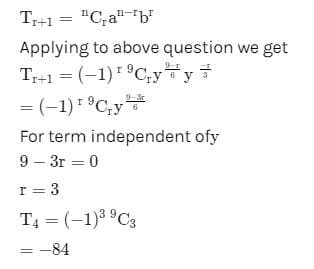BITSAT Maths Test - 2 - Question 3

### Equation of the diameter of the circle x2 + y2 - 6x + 2y = 0 which passes thro' the origin is

BITSAT Maths Test - 2 - Question 4

If the two circles 2x2 + 2y2 -3x + 6y + k = 0 and x2 + y2 - 4x + 10y + 16 = 0 cut orthogonally, then the value of k is

BITSAT Maths Test - 2 - Question 5

If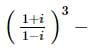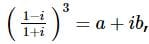then a and b are

BITSAT Maths Test - 2 - Question 6

The order and degree of differential equation √(dy/dx) - 4 (dy/ dx) - 7x = 0 are

Detailed Solution for BITSAT Maths Test - 2 - Question 6

√(dy/ dx)- 4 (dy/dx) -7x = 0
or √(dy/ dx) = 4 (dy/dx) + 7x
Squaring both side
dy/ dx = [4 (dy/dx ) + 7x]2
or dy dx = 16 ( dy/dx )2 + 49x2 + 56 dy/ dx x
∴ order = 1
degree = 2

BITSAT Maths Test - 2 - Question 7

If x dy = y(dx + y dy), y > 0 and y (1) = 1, then y (-3) is equal to

Detailed Solution for BITSAT Maths Test - 2 - Question 7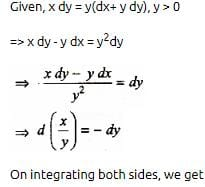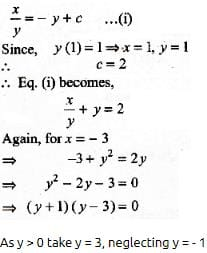BITSAT Maths Test - 2 - Question 8

Solution of the differential equation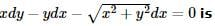BITSAT Maths Test - 2 - Question 9

If x=a[(cost)+(log tan(t/2))], y=a sint, (dy/dx)=

BITSAT Maths Test - 2 - Question 10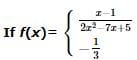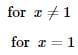then  = f '(1)

BITSAT Maths Test - 2 - Question 11

1/2! - 1/3! + 1/4! - 1/5! + ....... equals

BITSAT Maths Test - 2 - Question 12

The length of the shadow of a rod inclined at 10o to the vertical towards the sun is 2.05 metres when the elevation of the sun is 38o.The length of the rod is

BITSAT Maths Test - 2 - Question 13

The area of the region bounded by the curve y = x - x2 between x = 0 and x = 1 is

BITSAT Maths Test - 2 - Question 14

The product of the perpendicular, drawn from any point on a hyperbola to its asymptotes is

BITSAT Maths Test - 2 - Question 15

The sum of first 50 terms of the series cot⁻13 + cot⁻1 7 + cot⁻1 13 + cot⁻1 21 + ... is

BITSAT Maths Test - 2 - Question 16

The area bounded by the curve x = at2, y = 2at and the X-axis is 1 ≤ t ≤ 3 is

BITSAT Maths Test - 2 - Question 17

The domain of the function f(x) = √(2 - 2x - x2) is

BITSAT Maths Test - 2 - Question 18

The difference of an integer and its cube is divisible by

BITSAT Maths Test - 2 - Question 19

If A and B are two matrices such that AB = B and BA = A, then A2 + B2 is equal to

BITSAT Maths Test - 2 - Question 20

if A is a 3 x 3 matrix and B is its adjoint matrix. If ∣B∣ = 64, then ∣A∣ =

BITSAT Maths Test - 2 - Question 21

The strength of a beam varies as the product of its breadth b and square of its depth d. A beam cut out of a circular log of radius r would be strong when

Detailed Solution for BITSAT Maths Test - 2 - Question 21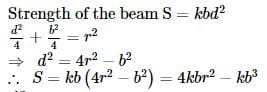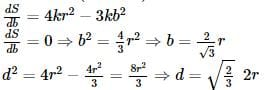BITSAT Maths Test - 2 - Question 22

Which of the following is correct

BITSAT Maths Test - 2 - Question 23

The pole of the line lx+my+n=0 w.r.t. the parabloa y2 =4ax

BITSAT Maths Test - 2 - Question 24

Equation x2+7xy+3y2+8x+14y+λ=0 represents a pair of straight lines, value of λ is

BITSAT Maths Test - 2 - Question 25

The latus rectum of the parabola y2 = 5x + 4y + 1 is

Detailed Solution for BITSAT Maths Test - 2 - Question 25

y2 = 5x + 4y + 1
or y2 - 4y = 5x + 1
or y2 - 2.2.y + (2)2 - (2)2 = 5x + 1
or (y - 2)2 = 5x + 5
or (y - 2)2 = 5(x + 1)
Length of the latus rectum = 5

BITSAT Maths Test - 2 - Question 26

How many total words can be formed from the letters of the word INSURANCE in which vowels are always together?

BITSAT Maths Test - 2 - Question 27

A polygon has 44 diagonals. The number of its sides is

Detailed Solution for BITSAT Maths Test - 2 - Question 27 The number of diagonals for n sided polygon = [n(n-3)]/2 . therefore, => 44 = [n(n-3)]/2 . => n^2 - 3n - 88 = 0 . => (n+8)(n-11) =0. => n = -8 or 11. Neglect n = -8. Therefore, => n = 11 . therefore, the no. of sides = 11 Hence, correct answer is (B).
BITSAT Maths Test - 2 - Question 28

A committee consists of 9 experts from three institutions A, B and C, of which 2 are from A, 3 from B and 4 from C. If three experts resign, then the probability that they belong to different institutions is

BITSAT Maths Test - 2 - Question 29

A single letter is selected at random from the word "PROBABILITY". The probability that it is a vowel is

BITSAT Maths Test - 2 - Question 30

If the area of a Δ A B C be λ then a2 sin 2B + b2 sin 2A is equal to

Detailed Solution for BITSAT Maths Test - 2 - Question 30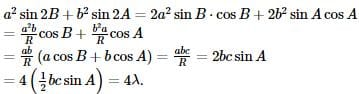BITSAT Maths Test - 2 - Question 31

In a Δ A B C , a = 1 and the perimeter is six times the AM of the sines of the angles. The measure of ∠ A is

Detailed Solution for BITSAT Maths Test - 2 - Question 31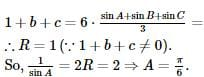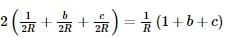BITSAT Maths Test - 2 - Question 32

If α, β are the roots of the equation x2- 2x + 2 = 0, then the value of α2 + β2 is

BITSAT Maths Test - 2 - Question 33

The sum of an infinite G.P. is 3. The sum of the series formed by squaring its terms is also 3. The series is

BITSAT Maths Test - 2 - Question 34

The 5th term of a G.P. is 2, then the product of its first 9 term is

BITSAT Maths Test - 2 - Question 35

If f : R → R is given as f (x) = |x| and A = {x ∈ R : x > o}, then f⁻1(A) =

BITSAT Maths Test - 2 - Question 36

If f (x) = {2x - 3, x ≤ 2} {x, x > 2} then f (1) is equal to

BITSAT Maths Test - 2 - Question 37

The equation line passing through the point P(1,2) whose portion cut by axes is bisected at P, is

Detailed Solution for BITSAT Maths Test - 2 - Question 37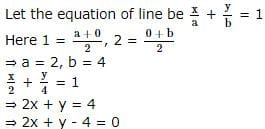BITSAT Maths Test - 2 - Question 38

The equation of the common tangent to the curves y2=8x and xy=-1 is :

BITSAT Maths Test - 2 - Question 39

The radius of the circle in which the sphere x2 + y2 + z2 + 2x - 2y - 4z - 19 = 0 is cut by the plane x + 2y + 2z + 7 = 0, is

Detailed Solution for BITSAT Maths Test - 2 - Question 39 Radius of sphere is=5 then perpen dicular dice Frome plane=4 now radius of circle r,sqr=25_16=9so r=3
BITSAT Maths Test - 2 - Question 40

The acute angle between the planes 2x-y+z=6 and x+y+2z=3 is

BITSAT Maths Test - 2 - Question 41

If 0≤x≤π and 81sin2x + 81 cos2x = 30, then x is equal to

BITSAT Maths Test - 2 - Question 42

If 1 + sin x + sin 2 x + … ∞ = 4 + 2 √ 3 , 0 < x < π , x ≠ π/2 then x =

BITSAT Maths Test - 2 - Question 43

(secA+tanA-1)(secA-tanA+1)-2 tanA=

BITSAT Maths Test - 2 - Question 44

The value of b such that the scalar product of the vector î+ĵ+k̂ with the unit vector parallel to the sum of the vectors 2î + 4ĵ - 5k̂ and bî+ 2ĵ + 3k̂ is one is

Detailed Solution for BITSAT Maths Test - 2 - Question 44

Parallel vector =(2+b)i+6j−2k

Unit vector = (2+b)i+6j−2k / (b2+4b+44)1/2

According to the question,

1 = (2+b)+6−2/b2+4b+44

⇒b2+4b+44=b2+12b+36

⇒8b=8⇒b=1

BITSAT Maths Test - 2 - Question 45

The angle between the vectors 3i+j+2k and 2i-2j+4k is

## BITSAT Mock Tests Series & Past Year Papers

2 videos|15 docs|70 tests
 Use Code STAYHOME200 and get INR 200 additional OFF Use Coupon Code
Information about BITSAT Maths Test - 2 Page
In this test you can find the Exam questions for BITSAT Maths Test - 2 solved & explained in the simplest way possible. Besides giving Questions and answers for BITSAT Maths Test - 2, EduRev gives you an ample number of Online tests for practice

## BITSAT Mock Tests Series & Past Year Papers

2 videos|15 docs|70 tests